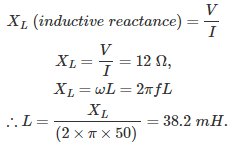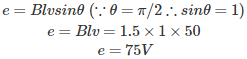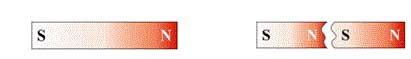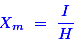# MCQs on Electric Circuits

##### Page 20 of 63. Go to page 1 2 3 4 5 6 7 8 9 10 11 12 13 14 15 16 17 18 19 20 21 22 23 24 25 26 27 28 29 30 31 32 33 34 35 36 37 38 39 40 41 42 43 44 45 46 47 48 49 50 51 52 53 54 55 56 57 58 59 60 61 62 63
01․ The coils having self inductor of 10 mH and 15 mH have an effective inductor of 40 mH, when connected in series aiding. What will be the equivalent inductor if we connect them in series opposing
20 mH.
5 mH.
0 mH.
10 mH.

L = L1 + L2 + 2M, when adding 40 = 10 + 15 + 2M 2M = 15 mH L = L1 + L2 - 2M , when opposing L = 10 mH.

02․ In an AC circuit, containing pure inductance, the voltage applied is 120 V, 50 Hz while the current is 10 A. Find the value of inductance.
35 mH.
34 mH.
30 mH.
38 mH.03․ A conductor of length l meters moves at right angles to a uniform magnetic field of flux density B = 1.5 T. if the velocity of revolution of the conductor is 50 ms-1 then induced e.m.f. in the conductor is
75 V.
0 V.
100 V.
125 V.04․ The stray line of magnetic flux is defined as
a line vertical to the flux line.
the mean length of a ring shaped coil.
the mean length of a ring shaped coil.
a line of magnetic flux that does not follow the designed path.

The stray magnetic field can cause an unwanted magnetic field between the magnetic source and the area where no stray magnetic field is required. The unwanted magnetic fields usually do not follow the designed path.

05․ A rectangular magnet of magnetic moment M is cut into two pieces of same length. The magnetic moment of each piece will be
M / 2.
2 M.
M.
M / L.As shown in the above figure, if we cut the magnet into two pieces of same length, then the magnetic moment M would also divide into two equal values. Hence, the new magnetic moment of each piece is M / 2.

06․ The ratio of intensity of magnetisation to the magnetising force is known as
relative permeability.
susceptibility.
flux density.
none of above.

The magnetic susceptibility indicates how easily the material can be magnetized. It is expressed by the following equation.Hence, it is the ratio of magnetization (I) induced in the material to magnetizing force (H). Therefore Xm is a number and has no units.

07․ Which of following is the unit of magnetic flux density?
Tesla.
Daraf.
Lumens.
Weber.

Nikola Tesla was best known for his contributions to the design of the modern alternating current electricity supply system and his name has been honored with an international unit of magnetic flux density. 1 Tesla = 1 Wb/m2.

08․ Which part of magnetic path requires largest mmf?
Air gap.
Coil.
Inductance.
Core.

The air gap is basically to make the magnetization characteristics of the circuit more linear. It ensures a linear relationship between voltage and current. Hence, the air gap allows more flux to flow in it. It requires largest mmf because it offer more reluctance than iron part.

09․ Emf induced in a coil rotating in a uniform magnetic field will be maximum when the
flux linking with the coil is maximum.
rate of change of flux linkage is minimum.
rate of change of flux linkage is maximum.
rate of cutting flux by coil sides is minimum.

The rate of change of flux linkage is directly proportional to the induced EMF in a coil. Hence, the EMF is maximum when the rate of change of flux linkage is maximum.

10․ A coil with negligible resistance has 50 V across it with 10 mA. The inductive reactance is
50 ohms.
500 ohms.
1000 ohms.
5000 ohms.

The inductive reactance XL = [ Voltage / Current] = 50 / (10 × 10-3) = 5000 Ohms.

<<<1819202122>>>